# The Mixing of Solids

| Home | | Pharmaceutical Technology |

## Chapter: Pharmaceutical Engineering: Mixing

Pharmacy offers many important examples of the mixing of solids. In several forms of drug presentation, the attainment of accurate dosage depends on an adequate mixing operation at some stage in production.

THE MIXING OF SOLIDS

Pharmacy offers many important examples of the mixing of solids. In several forms of drug presentation, the attainment of accurate dosage depends on an adequate mixing operation at some stage in production. Since the dose unit may be small, say 0.1 g, a small scale of scrutiny is applied.

The mixing of all systems of matter involves a relative displacement of the particles, whether they are molecules, globules, or small crystals, until a state of maximum disorder is created and a completely random arrangement is ach-ieved. Such an arrangement for a mixture of equal parts of two components is shown in Figure 13.1B.

A “perfect” mixture, which, with a practical sample, would offer point uniformity, is shown in Figure 13.1A. Such an arrangement is, however, virtu-ally impossible, and no mixing equipment can do better than producing the “random” mixture shown in Figure 13.1B. In such a mixture, the probability of finding one type of particle at any point in the mixture is equal to the proportion of that type of particle in the mixture.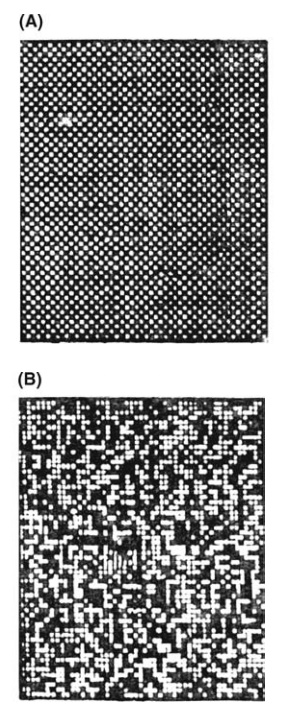FIGURE 13.1 Diagrammatic representation of (A) a perfect mix and (B) a random mix.

The mixing of solids differs from the mixing of liquids in that the smallest practical sample withdrawn from a mixture of two miscible liquids contains many millions of particles. In the mixing of solids, a small sample contains relatively few particles, and examination of Figure 13.1B should show that such samples will show considerable variation with respect to the overall composi-tion of the mixture and that this variation should be reduced as the number of particles in the sample is increased. Assessing the variation in, say, drug content in a series of samples drawn from a mixture of powders is of great importance. The tablet machine may be regarded as a volumetric sampling device, and the variation in drug content between one tablet and the next is largely controlled by the mixing stage that precedes it.

### Some Properties of a Random Mixture

“Lacey, 1953” showed that the variation in the composition of samples drawn from a random mixture of two materials could be expressed by the following relation: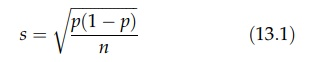where s is the standard deviation of the samples, p is the proportion of one component, and n is the number of particles in the sample. The relation requires that the two components are alike in particle size, shape, and density and can only be distinguished by some neutral property, such as color. If a very large number of samples, each containing a given number of particles, are withdrawn from a mixture of equal parts of two materials, the results of analysis can be presented in the form of a frequency curve in which the samples are normally distributed around the mean content of the mixture; 99.7% of the samples will fall within the limits p = 0.5 + 3σ. The standard deviation of the samples is inversely proportional to the square root of the number of particles in a sample. If the particle size is reduced so that the same weight of sample contains four times as many particles, the standard deviation will be halved. The distribution of samples and the effect of size reduction are shown in Figure 13.2.

In a critical examination of pharmaceutical mixing, Train showed that samples of a random mixture of equal parts of A and B must contain at least 800 particles if 997 out of every 1000 samples (3σ) were to lie between 10% of the stated composition, that is, the proportion, p, of A = 0.5 ± 0.05, where σ = 0.05/3 (Train, 1960). The effect of the number of particles in a sample on the percentage variation about the mean content of a mixture of equal parts A and B was summarized by Train in the diagram reproduced in Figure 13.3. The diagram may be used to show that if, in the above example, limits of ±1% were sub-stituted, 90,000 particles must be present in each sample. The true standard deviation is given by the symbol s. The standard deviation estimated by the withdrawal of a number of samples is denoted s.

If, instead of equal parts A and B, the proportion of an active ingredient, A, in the mixture was 0.1 (10%), imposition of limits of ±10% (in 997 out of 1000 cases) requires that each sample shall contain over 8000 particles. If the pro-portion of active constituent is 0.01, or 1%, a figure of 90,000 particles per sample is obtained, and if the limits are reduced to +1%, the active constituent is 0.01, or 1%, a figure of 90,000 particles per sample is obtained, and if the limits are reduced to ±1%, the figure is nine million.FIGURE 13.2 Distribution of samples drawn from a mixture of equal parts A and B. The broken line represents data for the coarser powder.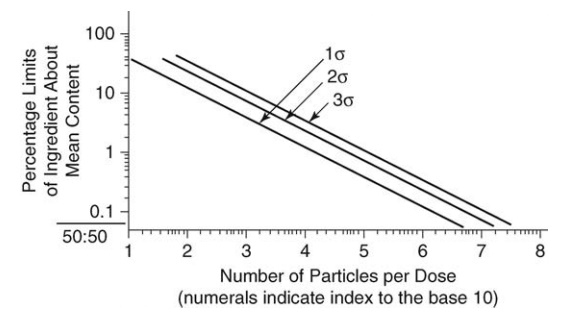FIGURE 13.3 General theoretical relationship between number of particles and percentage limit of ingredient for a 50:50 mix.

The theoretical derivation of these results is based on component particles that are alike in size, shape, and density. This condition is not encountered in the practical mixing of solids, and, as we shall see later, any of these factors may prevent the formation of a random mixture. The value of the number of particles per sample derived in any example must therefore be raised if the limits given are to be maintained.

As the proportion of an active ingredient in a mixture decreases, the number of particles in each sample or dose must increase, and materials of smaller particle size must be used. This statement indicates the limitation of the mixing of solids. Production of very fine powders is difficult and often attended by severe aggregation, thus defeating the object of size reduction in the mixing process. Where the proportion of active constituent is very small and is finally presented in a small dose unit, dry mixing of solids may fail to produce an adequate dispersion of one component in another and other meth-ods, such as spraying a solution of one component onto another, must then be adopted.

Another example of the relation of dose uniformity and number of particles in the dose is found with two components that are separately granu-lated before mixing. This procedure is sometimes adopted for reasons of sta-bility during granulation. The variation in samples drawn from such a system will be much greater than the variations in a mixture that was mixed before granulation because the effective number of particles in the sample is greatly reduced.

The Degree of Mixing

A quantitative expression that defines the state of a mix is necessary if a rational answer to the question “Is this material well enough mixed?” is to be made. Such an expression would also allow the course of mixing to be followed and the performance of different mixers to be compared. The most useful method of expressing the degree of mixing is by measuring the statistical variation in composition of a number of samples drawn from the mix. The scale of scrutiny determines the size of the samples, and their number depends on the accuracy required of the assessment.

As already shown, a series of samples drawn from a random mix exhibit a standard deviation of sr. An index of mixing, M, suggested by Lacey, is given by

M = sr/s                        (13.2)

where s is the standard deviation of samples drawn from the mixture under examination (Lacey, 1954). This approaches unity as mixing is completed. Kramers has suggested that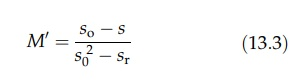where so is the standard deviation of samples drawn from the unmixed mate-rials (Shotton and Ridgway, 1974). It is equal to p(1 - p), where p is the pro-portion of the component in the mix. Its modification by Lacey, using the variance of the samples, togives a fundamental equation for expressing the state of the mixture, the index, M, varying from 0 to 1 (Lacey, 1954).

The binomial and Poisson distributions have also been used to examine the state of a mixture. If the proportion of black particles in a random mixture of black and white particles is p, the probability, P(x), of obtaining x black particles in a sample of n particles is given by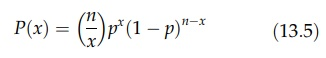If p is small (<0.15) and n is large, the Poisson distribution can be used, when

P(x) = e-m(mx/xl)               (13.6)

where m = np, the mean number of black particles in the samples of n particles. This relation may be used in an assessment of dry mixing equipment (Adams and Baker, 1956).

If m is greater than 20 and more than 10 samples are taken, then

1. about 10 of the samples will have the number of black particles outside the limits (m ± 1.7H√m),

2. about 5% of the samples will have the number of black particles outside the limits (m ± 2.0H√m), and

3. about 1% of the samples will have the number of black particles outside the limits (m ± 2.6H√m).

Results of such tests in which small cubes of polythene were mixed in a double-cone blender are shown in Figure 13.4A and B. The probability that the results plotted in Figure 13.4A came from a random mixture is less than 0.01, 19 out of 34 samples exceeding the 1 in 10 limits. The densities of the two com-ponents in this example were 0.92 and 1.2. The results given in Figure 13.4B were obtained when the components were of the same density and the proba-bility that the samples were drawn from a random mixture was 0.7.

Alternatively, satisfactory mixing may be established by imposing standards dictated by the operations in which the mixture is to take part. For example, Kaufman measured the variance of 10 samples drawn at random from a mixture of procaine penicillin and dihydrostreptomycin being mixed in a tumbler blender (Kaufman, 1962). The variance of the samples at different times during mixing is shown in Figure 13.5. The samples, which, in this case, weighed 5 g, represent the ultimate subdivision of a production-size antibiotic mixture.FIGURE 13.4 Variation in the number of black particles in samples drawn from a tumbler blender. (A) p < 0.01 and (B) p = 0.7.FIGURE 13.5 Decrease in the variance of samples drawn from a mixture of penicillin (40%) and dihydrostreptomycin in a twin-shell blender.

An acceptable degree of homogeneity was set at a standard deviation of 5%, giving a variance of (0.05)2, and this was achieved after just over 100 revolutions of the mixer. [The band around the experimental values of the variance defines the limits within which the true variance lies (p = 0.9)]. By this method, the suitability of the machine and operating characteristics were established.

The Mechanism of Mixing and Demixing

The randomization of particles by relative movement, one to another, is ach-ieved by the following mechanisms:

Convective mixing: The transfer of groups of adjacent particles from one location in the mass to another.

Diffusive mixing: The distribution of particles over a freshly developing surface.

Shear mixing: The setting up of slip planes within the mass.

All take place to some extent during mixing, but they vary in extent with the type of mixer used. In general, a large diffusional element is necessary if the scale of scrutiny is small. In addition, distortion of portions of the material by intense shear forces, as in mulling, and the scattering of individual particles by impact, processes normally associated with size reduction, are used for some mixing operations.

Convective mixing predominates in machines utilizing a mixing element moving in a stationary container. An example is the horizontal ribbon mixer. Groups of adjacent particles are moved from one position to another, giving a steady decrease in the scale of segregation.

Shear mixing occurs when a system of forces acting on the particles induce the formation of a slip place. This gives relative displacement of two regions. It occurs, for example, in the rearrangement of shape as the main charge falls from end to end in a double-cone mixer. Train has stressed the importance of expansion or dilation of the material so that shear forces may be effective (Train, 1960). A practical corollary is that efficiency will be reduced if the machine is overfilled.

Diffusive mixing predominates in tumbler mixers. Tumbling occurs as the material is lifted past its angle of repose. Mixing occurs when a particle changes its path of circulation through a collision or by being trapped in voids presented by another layer of particles.

The mild forces involved in the examples given above may be insufficient to adequately disperse materials that tend to aggregate. The more energetic pro-cesses of mulling and impact milling may then be used. Size reduction and mixing are carried out simultaneously, although the former may be slight. An example is found in the incorporation of ferric oxide and basic zinc carbonate in the pro-duction of calamine. For mixing of this type, the hammer mills, mullers, and ball mills charged with small balls are frequently used. The material being processed at any time must contain the correct amounts of materials. If the holdup capacity of the mill is sufficiently large, this can be achieved by a correctly proportioned feed. Otherwise, the product will have to be mixed a second time by some other method to correct segregation of large scale but small intensity.

If all the particles in a mixture reacted equally to an applied force, then all mixers would eventually produce a random mixture, although the time taken would vary, the more efficient mixer producing a random mix more quickly. The characteristics of real mixtures prevent this, and differences in particle size, shape, and density oppose randomization. Of these, differences in particle size are most important. The role of these factors in opposing mixing and promoting demixing is demonstrated in the analysis of horizontal drum mixing (Donald and Roseman, 1962). Movement of material in a radial plane is shown in Figure 13.6. The static mass of particles is lifted past its angle of repose, and particles tumble down the free surface, accelerating to the center of the mixer and then decelerating before entering the static bed. The zone in which this takes place is the mixing zone, and since it is in contact with the static bed, in which no mixing takes place and which is moving in the opposite direction, a velocity gradient occurs across the mixing zone, that is, a layer of particles is passing over the layer beneath, and so on. This zone is in an expanded state, and particles are therefore passing over voids in the layer beneath.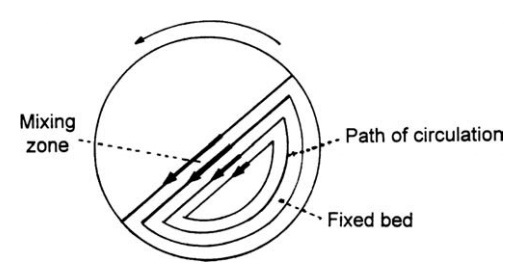FIGURE 13.6 The mechanism of radial mixing and demixing.

Mixing occurs when a particle is trapped by moving into a void, thus changing its path of circulation. This mechanism suggests an optimum running speed. If it is too slow, not enough events occur. If it is too fast, there is not sufficient time for capture.

As long as one type of particle is not preferentially caught, a random mix will eventually occur in the radial plane. If, however, one component is smaller or denser or has certain shape characteristics, it will be preferentially trapped and will move into the lower layers of the mixing zone until it finally concen-trates as a central core running the length of the mixer. Similar effects occur in axial mixing, and the final shape of the segregated zone formed under the influence of axial and radial movements depends on the flow properties of the material. Similar effects have been reported with a double-cone blender (Adams and Baker, 1956). Segregation will also occur with such materials when they are dumped from the mixer.

In general, one component will concentrate at one position in the mixer when a simple, repetitive, and symmetric movement occurs. Modern design tends to the rotation of asymmetric shapes or to symmetric shapes rotated asymmetrically, often with an abrupt reversal in the movement of the charge. Even so, segregation may still occur after a long period of mixing. The variance of samples decreases during mixing to a minimum value. This is followed by a period of demixing, the variance finally achieving a higher equilibrium value. It is therefore possible to overmix.

### The Rate of Mixing

Since mixing is a process of achieving uniform randomness, the rate of mixing is proportional to the amount of mixing still to be done. If, at the start of mixing, a particle changes its path of circulation, it is most likely to find itself in a different environment. The rate of mixing is therefore fast. At the end of the process, the particle is less likely to find a different environment, and such a change gives no useful mixing. Fewer mixing events will take place, and the rate of mixing finally reaches zero. The rate of mixing for any mixing mechanism can be rep-resented by the following expression:

dM/dt = k(1 – M)               (13.7)

where M, the index of mixing, has already been defined. Integration of this equation gives

M = 1 – e-kt               (13.8)

The rate constant, k, depends on the physical nature of the materials being mixed and on the geometry and operation of the mixer.